## Using Gabor functions to make atmosphere in computer graphics

This is a snapshot of some thoughts I've been having about a way to make highly detailed realistic computer graphic atmospheric effects that can run interactively on home computers. It's more mathematical than anything else here, but it seemed like a good idea to include at least one thing that's just talking about thinking about something.

Computer graphics realism in movies is greatly helped by atmospheric effects, such as mist, fog, and clouds. But you don't usually see really great atmosphere effects in real-time graphics on home computers. To understand why, it's useful to think about how 3D computer graphics images get made.

To make a 3D graphical image, a computer program describes an imaginary viewpoint, or "camera", which looks into the imaginary scene. At every point (pixel) of the image, the program figures out what object of the scene is visible from the camera at this pixel, and then what surface point of that object is visible. Finally, the program computes the color, texture and lighting at that surface point.

If you think about the physics of what's going on here, the thing that's being simulated is a ray of light travelling from the surface point of the object to the camera. "Atmosphere" is anything that influences that ray of light between the surface point and the camera (things like fog, mist, clouds).

So in order to properly render the appearance of atmosphere, you need to be able to answer the following question, at each pixel of the image: "If I follow this light ray from the visible surface point, back to the camera, how much light gets absorbed along the way?" Once you can answer this question, then you can also figure out how much the visible atmosphere (say, a cloud) casts shadows on the object. You do that by following the light ray from the light source to the surface point, and seeing how much light gets absorbed along that ray.

Since the 1970's, computer graphics programmers have used a very simple method for rendering a scene in the special case where a misty atmosphere has constant density everywhere. In that special case, we can measure the density of the atmosphere by how far light has to travel before half the light is absorbed or scattered away. Let's say, for example, that after traveling through some distance D of fog, a beam of light is attenuated by half. Then after going a further distance of D (a total distance of 2D, half of this remaining light will be gone, so that only 1/4 of the original light is left. After going a total distance of 3D, half of that light will be gone, so that only 1/8 of the original light is left.

We can write what we just said as a simple equation: After traveling any distance d, the light will be attenuated to 0.5d/D. We can say that the density of this fog is 1/D. For example, if the density of the fog is 0.1, then light gets halved after travelling a distance of 10 units. After travelling, say, 50 units, this light would be attenuated to 0.550/10 = 0.55 = 1/32.

What's nice about this simple case of uniform fog is that it doesn't matter where the ray of light starts or where it ends. All you need to measure is the total distance the light travels, in order to find out how much the light is attenuated. What we're actually computing is the line integral (ie: the integral of a function along a line) through the atmosphere. Line integrals are usually hard to compute, but not in this special case where density is constant everywhere.

The results get more interesting, and more difficult, if you put some structure into the atmosphere. In general, you'd like to be able to describe any arbitrary density function D(x,y,z), where (x,y,z) is a point in space, and D(x,y,z) is the density at that point. Then you want to ask the question: "Given any two points (Ax,Ay,Az) and (Bx,By,Bz) in space, what is the line integral of D(x,y,z) along the line segment that connects these two points?" Unfortunately, the line integrals for most functions are generally very difficult to compute.

Before going on, it's important to make a distinction between density and transparency. If you double density, then you square opacity. For example, if a density of D through a certain distance of fog produces a transparency of 0.5, then a density of 2D along the same distance produces a transparency of 0.25. You can see this with photographic filters. Say you have two filters, each of which knocks out half the light. If you place one filter in front of the other, thereby doubling the filter density, then you knock out first half the light, then half of what's left. If you stack three filters, then you end up with only 1/8 the transparency, and so on. In other words, transparency varies exponentially with density.

The most general (and slowest) way to compute the line integral is to march along each light ray, point by point. At each point you compute the density, and you sum together all those point densities. When you're done, you exponentiate this total density to compute the total transparency through the fog. This is how we made all those cool Hypertexture pictures. Unfortunately, this way of doing things is really expensive. For example, if you're rendering a 1000×1000 image, and you need to step an average of 1000 steps for each ray, you end up doing about a billion density computations. So you look for special cases.

In 1983 I came up with a simple way to make layered mist, while I was working at MAGI. Layered mist is the special case where the density varies only in one dimension (say, with height). We ended up using this a lot in the commercial work we did the year after we finished TRON, as we were trying to create more "naturalistic" graphics. It was used in the lovely award winning film "First Flight" by Tom Bisogno and Mike Ferraro, made in 1983 at MAGI (SIGGRAPH '84 Electronic Theater; SIGGRAPH Video Review library, Issue 15), in which a bird flies within a mountainous world through layers of mysterious mist.

The key to that special case was the observation that if density varies in only one dimension, then you can basically look at the whole thing as a one dimensional problem. Let's say that density only varies in the y dimension (vertically). If you march along a light ray point by point from (Ax,Ay,Az) to (Bx,By,Bz), the x and z coordinates at each point don't really matter, since density is the same for all values of x and z. So let's just set these both to zero. If you march vertically along the light ray (0,Ay,0) to (0,By,0), you get a similar result, except for not having to travel as far at each step along the ray.

Let's say you're marching along a ray, accumulating density, and the ray is slanted from the vertical by some angle θ. Then the ray will be elongated, compared to a vertical ray that travels the same vertical distance, by a factor of 1/cos(θ). That means that the density at every step of the integration must be greater by a factor 1/cos(θ), compared with a step along a vertical path. So the total density along the slanted ray (Ax,Ay,Az) to (Bx,By,Bz), the same as the total density along the vertical ray (0,Ay,0) to (0,By,0), multiplied by 1/cos(θ).

So here's the trick. Given any density function D that varies only with y, we precompute the integral E(y). Because this integral is just one dimensional, we can store an approximation of it in a one dimensional table. For example, suppose we're trying to make a mist layer, and we know that the density of the layer is always zero below the bottom of the layer (say, when y < y0), and above the top of the layer (say, above y > y1). We can make an array containing sampled values of E(y) between y0 and y1.

What we're really storing in E(y) is the indefinite integral, which is what you get if you sum up values starting at y = -∞. But we've already decided that all the values of D(y) are zero, for y < y0, so those values won't add anything to the integral. The great thing about storing a table of indefinite integrals is that it makes it very easy to find what we really want, the definite integral between any two yA and yB. All you need to do is subtract: E(yB) - E(yA).

So all you need to do at each pixel, to compute the density of layered fog, is subtract two values of E(y), and then multiply by 1/cos(θ), where θ is the angle that the light ray is slanted from the vertical. Once you have the density, you just compute 0.5density to get the fog's transparency, and use this transparency to blend in a fog color (eg: white). If you use this approach, you can get results like the below image, which was created a few years ago using a computer graphics rendering package that implements layered fog.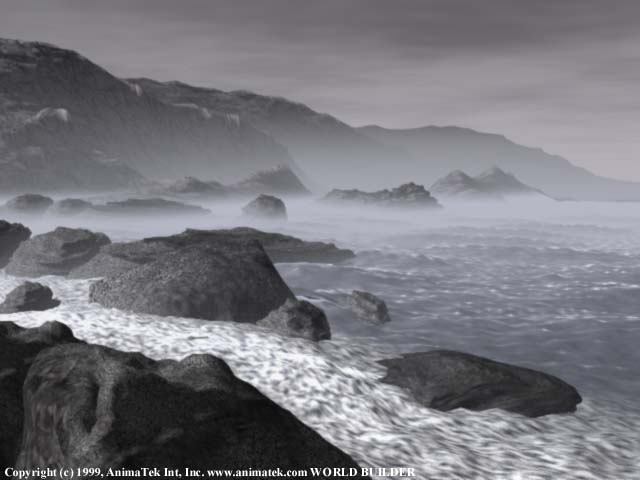Another kind of special case is cloudy things that are made up only of Gaussian functions. A Gaussian function is shaped as e-x2/2σ where e = 2.71828... is the natural base of logarithms.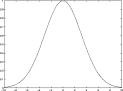The variable σ controls the width of the function. Gaussian functions make blurs. For example, here is a two dimensional Gaussian function e-(x2+y2)/2σ which creates a two dimensional blurry spot: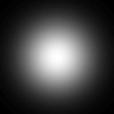It's easy to see that you could also create a three dimensional soft spherical cloud by addding the third, z, dimension: e-(x2+y2+z2)/2σ

One great thing about Gaussian density functions is that the density function along any line through them is also a Gaussian function. What this means for our purposes is that if you can integrate a Gaussian function in one dimension, then you can always find the cumulative density of a three dimensional Gaussian shaped cloud along any ray of light. You can also squeeze or stretch or flatten the fuzzy Gaussian ball into a pill or pancake shape, and you'll still get a Gaussian density function along any light ray.

The reason people don't often use Gaussians to make atmosphere in interactive computer graphics is that Gaussians are too visually simple, so you would need to use lots of them together to convey the appearance of irregularity that we associate with real clouds. And using lots of them together gets expensive. That's why you can see the approach of "hundreds of blurry spots to make atmosphere" in movies (where it's ok to take hours to compute a single image), but not for interactive graphics on home computers.

Recently I have been playing with something much more useful: the Gabor functions, which are Gaussian functions times (possibly phase-shifted) sine functions. These were discovered by Denis Gabor, who also invented Holography, as well as the phrase "The future cannot be predicted, but it can be invented." Below is a picture of a two dimensional Gabor function, which is a two dimensional Gaussian function times a (possibly phase-shifted) sine function: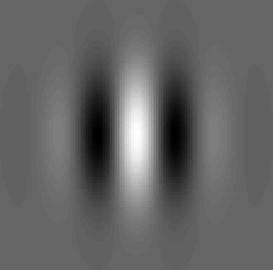Similarly, here is a picture of a three dimensional Gabor function, which is a three dimensional Gaussian function times a (possibly phase-shifted) sine function (see image below). One interesting thing about Gabor functions is that a line through a Gabor function is also a Gabor function. This is very good news if you're looking for things that can be integrated.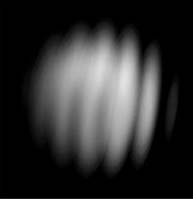It is easy to see that the density function along any line in space through a three dimensional Gabor function is a (one dimensional) Gabor function. Recall that a Gabor function is a product of two functions (a Gaussian function and a sine function). We already know that a line through a three dimensional Gaussian function is a Gaussian function. Also, a line that slants through a sine function is also a sine function, although generally a lower frequency (slower varying) one, since the more angled that the line is from the direction the sine wave propagates, the slower the sine waves will undulate as a function of distance along the line. Therefore the line will encounter a product of a Gaussian function and a sine function, which is a Gabor function!

So there you have it. I'm currently working on a way to use this approach to make complex interesting atmospheres that are made by summing up Gabor functions. By computing the line integrals on Graphics Accelarator boards, I hope to be able to make really interesting atmospheric shapes that consist of hundreds of Gabor functions and that can run interactively on home computers.